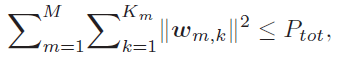# How to get the sum of square of norm of n-d matrix in CVX?

Hello Everyone,
I tried following equation to get sum of square of norm of n-d matrix in CVX:

size(w) = 6 1 2 7
sum(sum(square_pos(norms(w))))

using inner loop

for i = 1:2
for j = 1:6
a = a + square_pos(norm(w(:,:,i,j)))
end
end

In Matlab it works, but in CVX it is not able to do the outer sum. While applying loop (w(:,:,i,j)) also, inner sum was only done but unable to do the outer sum. Please guide me on this. Thank you for your help.

I’m not clear what actual algebraic formula you want. If you want the sum of squares of all elements, this should work

``````variable w(3,2,5,4)
sum(sum(sum(sum(w.^2))))
``````

You can perhaps make it a little faster by using the mathematically equivalent
`sum(sum(sum(sum_square(w))))` instead of `sum(sum(sum(sum(w.^2))))`

If you want something different (for instance, an actual matrix 2-norm applied to 2D slices of he variable), please clarify.

You can also look at `help sum_square` and `help norms` to see how N-D arrays are handled.

I want to achieve as given equation:w_{m,k} is a (N*1) matrix. Can you guide me on this?

Either of the formulations in my previous post should work. If you want a formulation which perhaps seems to correspond more closely to your image, you could use the also equivalent formula, `sum(sum(sum(square_pos(norms(w)))))`.

My guess is that `sum(sum(sum(sum_square(w))))` is the fastest executing of the formulations in terms of CVX processing time.

If your 2nd dimension is always 1, as you have indicated, you could make formulas simpler by eliminating that dimension in the variable declaration, and using one less `sum()` in the formula.

Thanks @Erling . I should have thought of just using `w(:) ` and avoiding all those sums, and then of course the possibly unnecessary squaring.